Ex 3.3

Chapter 3 Class 10 Pair of Linear Equations in Two Variables (Term 1)
Serial order wise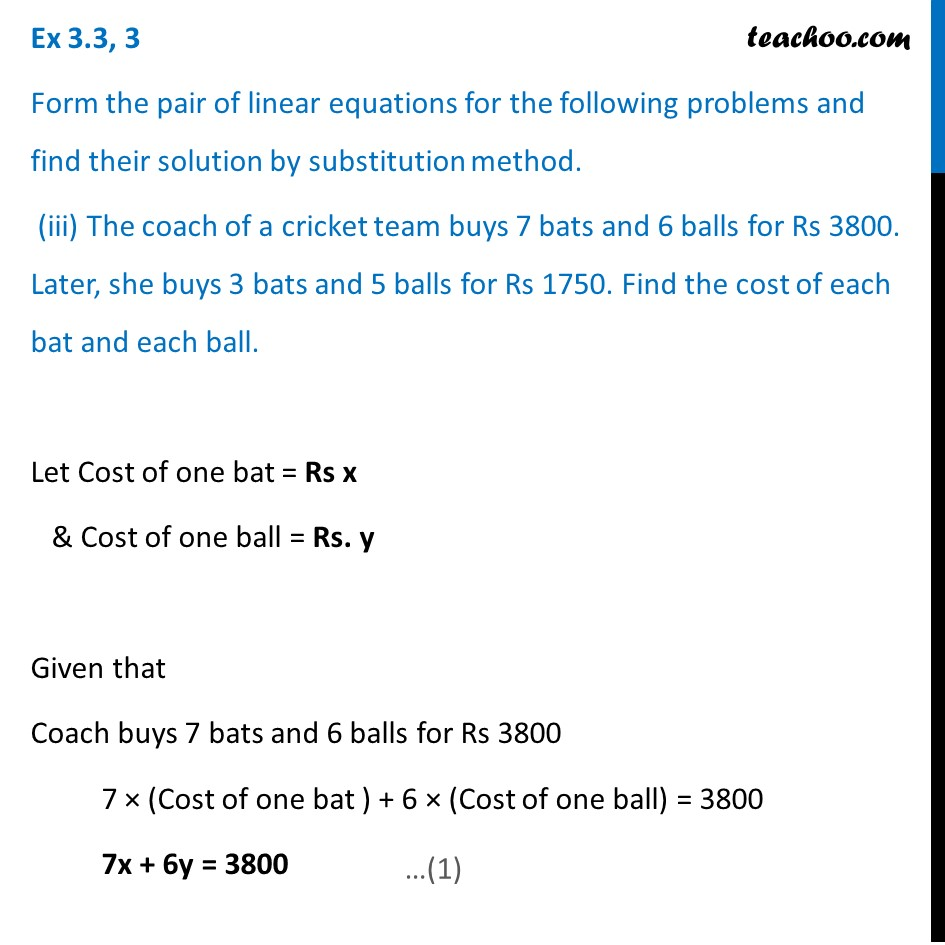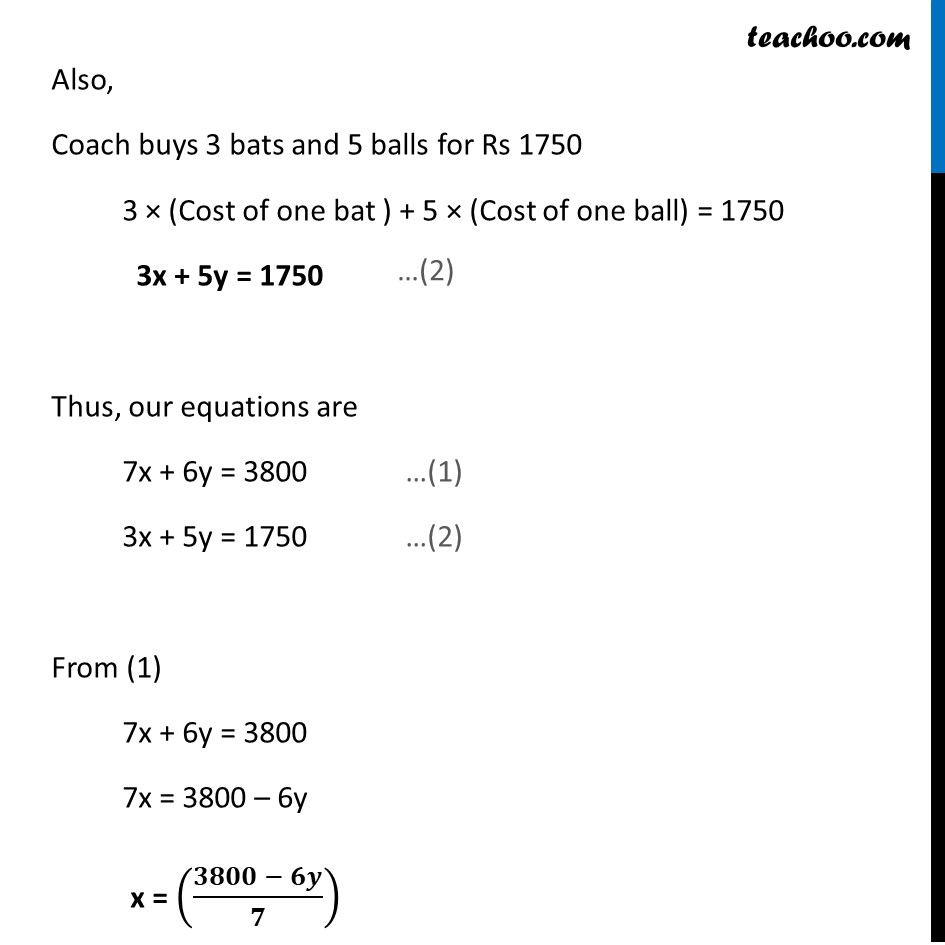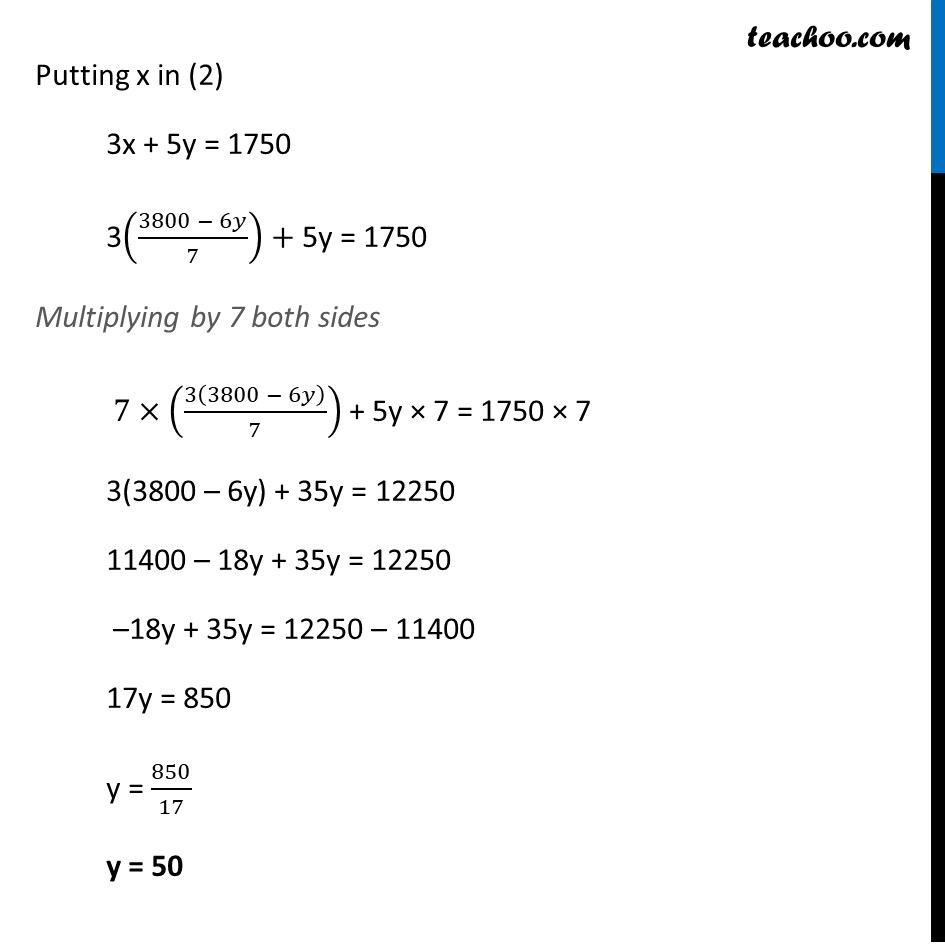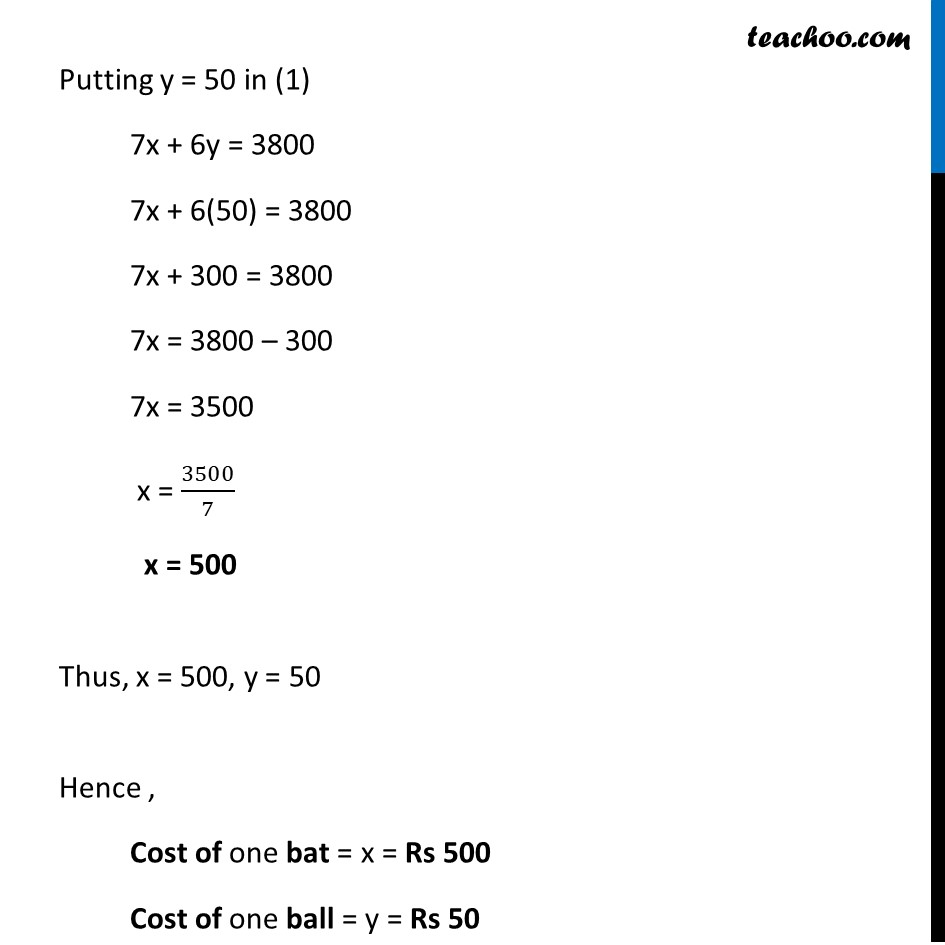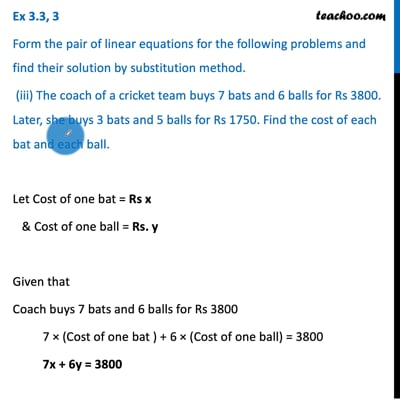This video is only available for Teachoo black users

### Transcript

Ex 3.3, 3 Form the pair of linear equations for the following problems and find their solution by substitution method. (iii) The coach of a cricket team buys 7 bats and 6 balls for Rs 3800. Later, she buys 3 bats and 5 balls for Rs 1750. Find the cost of each bat and each ball. Let Cost of one bat = Rs x & Cost of one ball = Rs. y Given that Coach buys 7 bats and 6 balls for Rs 3800 7 × (Cost of one bat ) + 6 × (Cost of one ball) = 3800 7x + 6y = 3800 Also, Coach buys 3 bats and 5 balls for Rs 1750 3 × (Cost of one bat ) + 5 × (Cost of one ball) = 1750 3x + 5y = 1750 Thus, our equations are 7x + 6y = 3800 …(1) 3x + 5y = 1750 …(2) From (1) 7x + 6y = 3800 7x = 3800 – 6y x = ((𝟑𝟖𝟎𝟎 − 𝟔𝒚)/𝟕) Putting x in (2) 3x + 5y = 1750 3((3800 − 6𝑦)/7)+ 5y = 1750 Multiplying by 7 both sides 7×(3(3800 − 6𝑦)/7) + 5y × 7 = 1750 × 7 3(3800 – 6y) + 35y = 12250 11400 – 18y + 35y = 12250 –18y + 35y = 12250 – 11400 17y = 850 y = 850/17 y = 50 Putting y = 50 in (1) 7x + 6y = 3800 7x + 6(50) = 3800 7x + 300 = 3800 7x = 3800 – 300 7x = 3500 x = 3500/7 x = 500 Thus, x = 500, y = 50 Hence , Cost of one bat = x = Rs 500 Cost of one ball = y = Rs 50